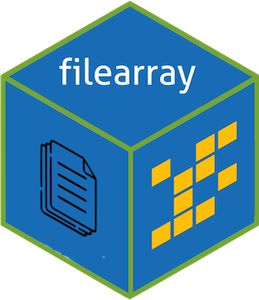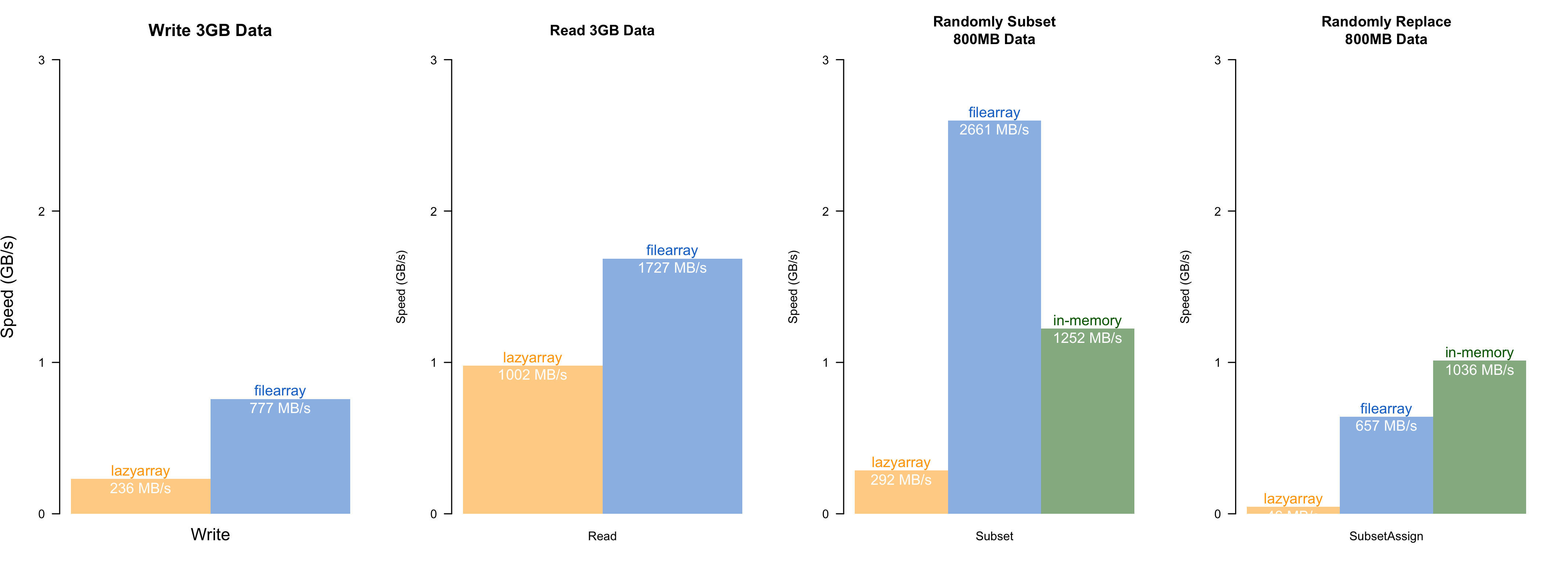Stores large arrays in files to avoid occupying large memories. Implemented with super fast gigabyte-level multi-threaded reading/writing via OpenMP. Supports multiple non-character data types (double, float, integer, complex, logical and raw).Speed comparisons with lazyarray (zstd-compressed out-of-memory array), and in-memory operation. The speed test was conducted on an MacBook Air (M1, 2020, 8GB RAM). filearray is uniformly faster than lazyarray. Random access has almost the same speed as the native in-memory operation.

## Installation

install.packages("filearray")

### Install Develop Version

The internal functions are written in C++. To avoid compiling the packages, you can install from my personal repository. It’s automatically updated every hour. Currently available on Windows and osx (Intel chip) only.

options(repos = c(
dipterix = 'https://dipterix.r-universe.dev',
CRAN = 'https://cloud.r-project.org'))

install.packages('filearray')

Alternatively, you can compile from Github repository. This requires proper compilers (rtools on windows, or xcode-select --install on osx, or build-essentials on linux).

# install.packages("remotes")
remotes::install_github("dipterix/filearray")

## Basic Usage

library(filearray)
file <- tempfile()
x <- filearray_create(file, c(100, 100, 100, 100))

x <- filearray_load(file)

See more: help("filearray")

#### Assign & subset array

x[,,,1] <- rnorm(1e6)
x[1:10,1,1,1]

#### Generics

typeof(x)
max(x, na.rm = TRUE)
apply(x, 3, min, na.rm = TRUE)

val = x[1,1,5,1]
fwhich(x, val, arr.ind = TRUE)

See more: help("S3-filearray"), help("fwhich")

#### Map-reduce

Process segments of array and reduce to save memories.

# Identical to sum(x, na.rm = TRUE)
mapreduce(x,
map = \(data){ sum(data, na.rm = TRUE) },
reduce = \(mapped){ do.call(sum, mapped) })

See more: help("mapreduce")

#### Collapse

Transform data, and collapse (calculate sum or mean) along margins.

a <- x\$collapse(keep = 4, method = "mean", transform = "asis")

# equivalent to
b <- apply(x[], 4, mean)

a - b

Available transform for double/integer numbers are:

• asis: no transform
• 10log10: 10 * log10(v)
• square: v * v
• sqrt: sqrt(v)

For complex numbers, transform is a little bit different:

• asis: no transform
• 10log10: 10 * log10(|x|^2) (power to decibel unit)
• square: |x|^2
• sqrt: |x| (modulus)
• normalize: x / |x| (unit length)